# Change Nickname

Current Nickname:

Certifications

Certificate Issued at Valid until Serial No. File
Serial No. Valid until

Not having one? Apply now! >>>

Invoice

ProductName CreateTime ID Price File
ProductName CreateTime ID Price File

# Connected Component

## Overview

Connected Component algorithm is used to count the number of connected components in the current GraphSet, it is an indicator to examine the connectivity of the graph.

Connected component is a coarse-grained metering method, if the number of connected components of a graph does not change, it can be considered from a macroscopic point of view that the topology characteristics of the graph have not changed.

## Basic Concept

### Connected Graph

In undirected graph, if there is path between any two nodes, the graph is regarded as connected. There is no path between the red and green nodes in the graph below, so it is not connected.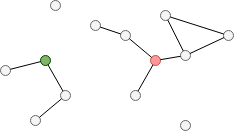In directed graph, if there is path between any two nodes in at least one direction, the graph is regarded as Weakly Connected Graph; if there are paths in both two directions, the graph is regarded as Strongly Connected Graph. Known from the definition, strongly connected graph must meet the conditions of weakly connected graph.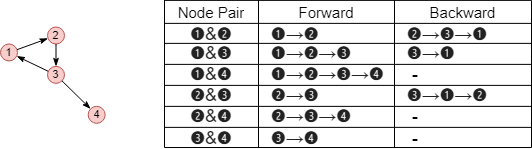In the graph above, node pairs `(1,4)`, `(2,4)` and `(3,4)` only have one-way paths, the graph only meets the requirements of weakly connected graph. Let's add another edge to the graph and calculate again: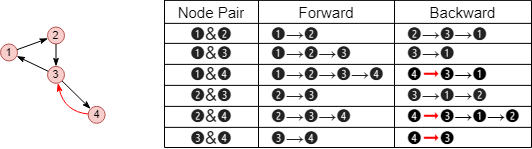After the modification, all node pairs in the graph have bidirectional paths, so the modified graph is strongly connected graph.

The criterion for determining a weakly connected graph in a directed graph can also be understood as: see if there is path between any two nodes when ignoring the direction of edges in the graph.

### Connected Component

A connected component is a connected subgraph that is not part of any larger connected subgraph. According to the definition of connected graph, connected component can be divided into Weakly Connected Component and Strongly Connected Component.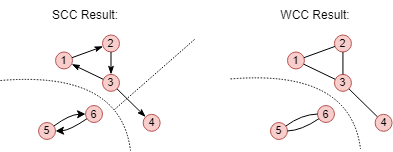The example above calculates the strongly and weakly connected components of the same graph. The graph can be divided into 3 strongly connected components, or 2 weakly connected components. Strongly connected component has more stringent judgement conditions than weakly connected component, so that the number of strongly connected components is always greater than the number of weakly connected components.

## Special Case

### Lonely Node, Disconnected Graph

Each lonely node in the graph is an independent connected component, it is both a strongly connected component and a weakly connected component.

This algorithm calculates the number of connected components, if the current graph is a strongly connected graph, it has 1 connect component.

### Self-loop Edge

Self-loop edge does not affect the connectivity of the graph, i.e. it does not participate in the calculation of connected components.

### Directed Edge

Calculation of strongly connected component relies on the direction of directed edges, calculation of weakly connected component ignores the direction of edges.

## Results and Statistics

Take the graph below as an example, run the Connected Component algorithm against all nodes: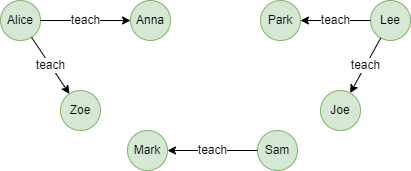Algorithm results 1: Calculate weakly connect components of the whole graph, return `_id`, `community_id` or `_uuid``community_id` according to the execution method

_uuid _id community_id
8 Sam 0
7 Mark 0
2 Anna 2
1 Alice 2
3 Zoe 2
4 Lee 5
5 Park 5
6 Joe 5

Algorithm statistics 1: Number of weakly connected components `community_count`

community_count
3

Algorithm results 2: Calculate strongly connect components of the whole graph, return `_id`, `community_id` or `_uuid``community_id` according to the execution method

_uuid _id community_id
8 Sam 0
7 Mark 0
2 Anna 2
1 Alice 3
3 Zoe 4
4 Lee 5
5 Park 6
6 Joe 7

Algorithm statistics 2: Number of strongly connected components `community_count`

community_count
7

## Command and Configuration

• Command: `algo(connected_component)`
• Configurations for the parameter `params()`:
Name
Type
Default
Specification
Description
cc_type int 1 1 or 2 1 or if not sets means to calculate weakly connected components (WCC), 2 means to calculate strongly connected components (SCC)
limit int -1 >=-1 Number of results to return; return all results if sets to -1 or not set
order string / ASC/DESC, case insensitive To sort the returned results; no sorting is applied if not set

Example: Calculate weakly connected components of the whole graph

``````algo(connected_component).params({
cc_type: 1
}) as wcc
return wcc
``````

## Algorithm Execution

#### 1. File Writeback

Configuration Data in Each Row
filename_community_id `_id`,`community_id`
filename_ids `community_id`,`_id`,`_id`,...
filename_num `community_id`,`count`

Example: Calculate strongly connected components of the whole graph, write the algorithm results back to file named info, members and count

``````algo(connected_component).params({
cc_type: 2
}).write({
file:{
filename_community_id: "info",
filename_ids: "members",
filename_num: "count"
}
})
``````

#### 2. Property Writeback

Configuration Writeback Content Type Data Type
property `community_id` Node property `int64`

Example: Calculate strongly connected components of the whole graph, write the community ID back to node property named community_id

``````algo(connected_component).params({
cc_type: 2
}).write({
db:{
property: "community_id"
}
})
``````

#### 3. Statistics Writeback

Name Data Type Description
`community_count` int Number of communities

Example: Calculate strongly connected components of the whole graph, write the algorithm statistics back to task information

``````algo(connected_component).params({
cc_type: 2
}).write()
``````

### Direct Return

Alias Ordinal Type Description Column Name
0 []perNode Node and its community ID `_uuid`, `community_id`
1 KV Number of communities `community_count`

Example: Calculate strongly connected components of the whole graph, define algorithm results and statistics as aliases named r1 and r2, and return the results and statistics

``````algo(connected_component).params({
cc_type: 2
}) as r1, r2
return r1, r2
``````

### Streaming Return

Configurations for the parameter `stream()`:

Name
Type
Default
Specification
Description
mode int 1 1 or 2 1 or if not sets means to return the community ID of each node, 2 means to return the number of nodes of each community
Alias Ordinal Type Description Column Name
0 []perNode / []perCommunity Node and its community ID / Community and its number of nodes `_uuid`, `community_id` / `community_id`, `count`

Example: Calculate weakly connected components of the whole graph, define algorithm results as alias named communities, and return the results ordered by the descending count of nodes in community

``````algo(connected_component).params({
order: "desc"
}).stream({
mode: 2
}) as communities
return communities
``````

### Real-time Statistics

Alias Ordinal Type Description Column Name
0 KV Number of communities `community_count`

Example: Calculate weakly connected components of the whole graph, define algorithm statistics as alias named count, and return the statistics

``````algo(connected_component).params().stats() as count
return count
``````